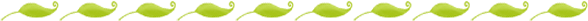# Subtraction Worksheets

Subtraction is a crucial math skill. It is important that students understand that subtraction involves taking away or pulling sets apart. As they grasp the underlying concept of subtraction, students can solve problems and begin to learn the subtraction facts.

The materials in this packet progress from worksheets that provide support for young

mathematicians to worksheets that challenge students to independently solve problems.  And fulfills the Common Core Standard CCSS.Math.Content.K.OA.A.5 Fluently add and subtract within 5.